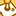# New to Qlik Sense

If you’re new to Qlik Sense, start with this Discussion Board and get up-to-speed quickly.

Announcements
QlikWorld starts MONDAY! last chance to register is now ! REGISTER NOW
cancel
Showing results for
Did you mean:Contributor III

## Nil field despite values below in sub-levels

Hello,

I have a report that has been working well with just "Master Account...", "StockCode" & "Name" as options in the pivot table. As soon as I added "SalesCategory" I found a strange issue that I cannot solve.  Under the Rebates & Discounts column the total for "SalesCategory" is nil despite everything below is calculating fine.The fields under E-Commerce and Gross sales have been blanked out for posting but are populated correctly with values. This includes the one rebates value that is blanked out too.

What could be causing this issue?

Thanks

Labels (1)
• ### SaaS

1 Solution

Accepted SolutionsMVP

How about if you try this

``````(Sum({\$<CalDate = {">=\$(=date(AddMonths(today(),-12)))<=\$(=date(today()))"}>} -[Gross sales value] * Rebate))
+
(Sum({\$<CalDate = {">=\$(=date(AddMonths(today(),-12)))<=\$(=date(today()))"}>} -[Gross sales value] * Discount))``````
4 RepliesMVP

What is your expression for Rebates & Discounts?Contributor III
Author

(Sum({\$ < CalDate = {">=\$(=date(AddMonths(today(),-12)))<=\$(=date(today()))"} > }-[Gross sales value])*Rebate)
+
(Sum({\$ < CalDate = {">=\$(=date(AddMonths(today(),-12)))<=\$(=date(today()))"} > }-[Gross sales value])*Discount)MVP

How about if you try this

``````(Sum({\$<CalDate = {">=\$(=date(AddMonths(today(),-12)))<=\$(=date(today()))"}>} -[Gross sales value] * Rebate))
+
(Sum({\$<CalDate = {">=\$(=date(AddMonths(today(),-12)))<=\$(=date(today()))"}>} -[Gross sales value] * Discount))``````Contributor III
Author

That's solved it. Thank you for this solution.